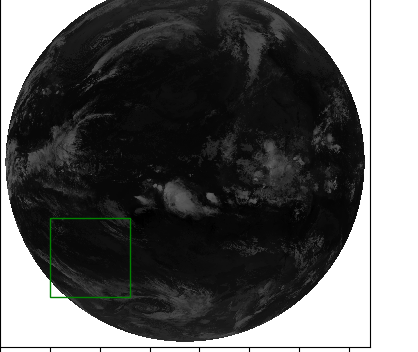# how mouve rectangle patch with mousehi,

I have a code example that allows to move the rectangle patch with the mouse every time I move the mouse somewhere on the graph I would like to use this example on an image and do the same thing add and move the ractangle with the mouse here I add the rectangle on the image but I can not move it with the mouse

the first code is example to move rectangle and the second code is my code i add rectangle on image

i need some help how can i move rectangle like example in my image :

import matplotlib.pyplot as plt
import matplotlib.patches as patches

def on_press(event):
xpress, ypress = event.xdata, event.ydata
w = rect.get_width()
h = rect.get_height()
rect.set_xy((xpress-w/2, ypress-h/2))

``````ax.lines = []
ax.axvline(xpress, c='b')
ax.axhline(ypress, c='b')

fig.canvas.draw()
``````

x = y = 1

fig = plt.figure()
fig.canvas.mpl_connect(‘button_press_event’, on_press)

rect = patches.Rectangle((x,y), 0.09,0.09, alpha=1, fill=None, label=‘Label’)

plt.show()

and that my exemple code draw rectangle on image :

import numpy as np
import netCDF4
from netCDF4 import Dataset
import matplotlib.pyplot as plt
import matplotlib.patches as patches

def on_press(event):
xpress, ypress = event.xdata, event.ydata
w = rect.get_width()
h = rect.get_height()
rect.set_xy((xpress-w/2, ypress-h/2))

``````ax.lines = []
ax.axvline(xpress, c='b')
ax.axhline(ypress, c='b')

fig.canvas.draw()
``````

fic=‘air_201905080000.nc’

path=’/home/data/’

nc = netCDF4.Dataset(path+fic,‘r’)
IR=nc.variables['IR][:]
x = y = 500

# Display the image

axes = plt.subplot(111)
axes.imshow(IR,origin=‘lower’, cmap=plt.cm.gist_yarg)

rect = patches.Rectangle((x,y),800,800,linewidth=1,edgecolor=‘g’,facecolor=‘none’)